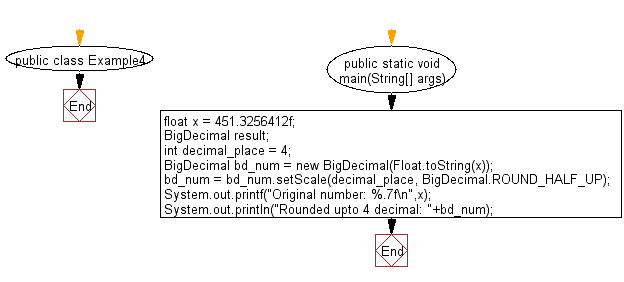﻿ Java Math Exercises: Round a float number to specified decimals - w3resource# Java Math Exercises: Round a float number to specified decimals

## Java Math Exercises: Exercise-4 with Solution

Write a Java program to round a float number to specified decimals.

Sample Solution:

Java Code:

``````import java.lang.*;
import java.math.BigDecimal;
public class Example4 {
public static void main(String[] args) {
float x = 451.3256412f;
BigDecimal result;
int decimal_place = 4;
BigDecimal bd_num = new BigDecimal(Float.toString(x));
bd_num = bd_num.setScale(decimal_place, BigDecimal.ROUND_HALF_UP);
System.out.printf("Original number: %.7f\n",x);
System.out.println("Rounded upto 4 decimal: "+bd_num);
}
}
``````

Sample Output:

```Original number: 451.3256531
Rounded upto 4 decimal: 451.3257
```

Flowchart:Java Code Editor:

What is the difficulty level of this exercise?

﻿

## Java: Tips of the Day

Parsing dates:

```import java.io.*;
import java.util.*;
import java.text.*;

String s = "2001/09/23 14:39";

SimpleDateFormat formatter = new SimpleDateFormat ("yyyy/MM/dd H:mm");
Date d = formatter.parse(s, new ParsePosition(0));
```